## 1. 学习环境

• 操作系统 Ubuntu Kylin 15.04

• 辅助软件 Eclipse Mars Release (4.5.0)

## 2. 安装JavaCC

### 2.1 在Eclipse中安装JavaCC插件

#### 2.1.1 下载JavaCC插件#### 2.1.2 试用JavaCC插件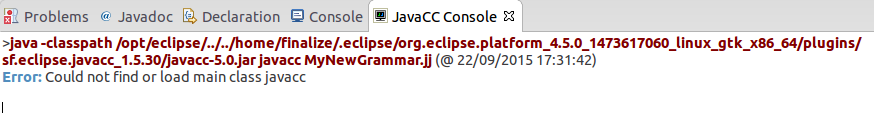#### 2.1.3 修复JavaCC插件错误配置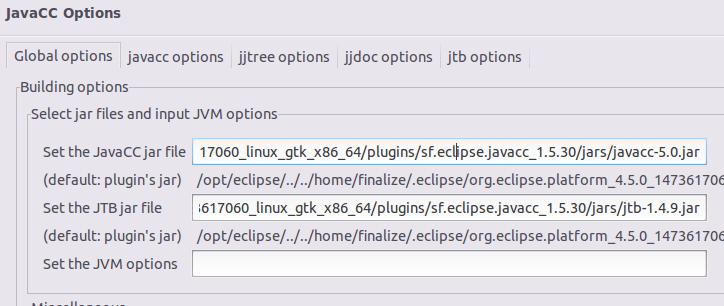### 2.2 安装独立Jar包

#### 2.2.1 下载独立Jar包

JavaCC官网下载java-6.0.zip。压缩包内包含独立Jar包以及JavaCC文档及示例程序。

#### 2.2.2 将Jar包添加到系统CLASSPATH

```export CLASSPATH=.:\$JAVA_HOME/lib/dt.jar:\$JAVA_HOME/lib/tools.jar:\$JAVA_HOME/lib/javacc.jar
```

#### 2.2.3 创建shell script，简化运行命令

```#!/bin/sh
java javacc \$*
```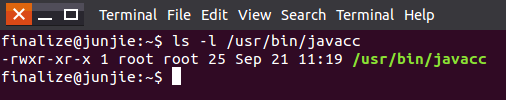#### 2.2.4 键入命令，运行javacc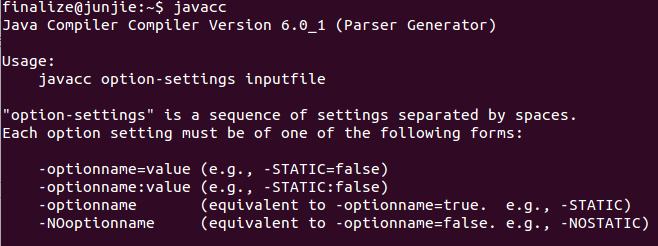#### 2.2.5 Debian/Ubuntu系统下安装

Debian/Ubuntu系统下，软件源中已存在javacc,使用如下命令即可完成安装

```sudo apt-get install javacc
```

### 3. JavaCC(Java Compiler Compiler)简介

• javacc 根据模板文件生成语法分析器
• jjtree 根据模板文件生成语法生成树
• jjdoc 根据模板文件生成对应语言的文档

• 输入：一些以正则表达式表示的词法单元，还有一些使用这些词法单元的语法规则
• 输出：一个词法分析器：读取输入并将其拆分为词法单元(Token)；一个语法分析器，分析输入的字符串是否满足定义的语法规则
• JavaCC文件格式为`MyParser.jj`
• `jj`文件通过命令`javacc MyParser.jj`进行处理
• 处理之后，会生成以下文件：
• `MyParser.java`：生成的语法分析器
• `MyParserTokenManager.java`：生成的词法分析器
• `MyParserConstants.java`：一些常量

Demo.jjt --> Demo.jj --> Demo.java --> Demo.class --> execute

### 4. javacc学习

javacc通过解析`.jj`的模板文件，生成自上而下的语法分析器。在默认情况下，javacc生成`LL(1)`语法分析器，用户可以自定义`LookAhead`的值，使其分析`LL(k)`语法。

#### 4.1 javacc语法

```javacc_input    ::= javacc_options
"PARSER_BEGIN" "(" <IDENTIFIER> ")"
java_compilation_unit
"PARSER_END" "(" <IDENTIFIER> ")"
( production )*
<EOF>
```

• "LOOKAHEAD" "=" java_integer_literal ";" 设置向前看的值，默认为1,对应于LL(1)文法
• "DEBUG_PARSER" "=" java_boolean_literal ";" 输出语法分析调试信息，默认为false
• "DEBUG_TOKEN_MANAGER" "=" java_boolean_literal ";" 输出词法分析调试信息，默认为false
• "IGNORE_CASE" "=" java_boolean_literal ";" 词法分析忽略大小写，默认为false
• "OUTPUT_DIRECTORY" "=" java_string_literal ";" 生成的java源文件的存放目录，默认为当前工作目录

`PARSER_BEGIN``PARSER_END`之间的部分为java代码，将会被复制到生成的主程序中，其中`IDENTIFIER`为生成的主程序类名，因此`java_compilation_unit`中的类声明应与之相同。

```production  ::= javacode_production
|   regular_expr_production
|   bnf_production
|   token_manager_decls
```

#### 4.2.1 示例1：Java注释解析

java的注释分为单行注释`//`与多行注释(包含文档注释)`/*....*/`，其中多行注释不允许嵌套，即形如`/*aaa/*bbb*/ccc*/`的形式是不合法的。下面将编写jj文件，描述其文法规则，并生成分析程序。

```options { static = true; }

public static void main(String args []) throws ParseException {
while (true){
System.out.print("Please input a java comment ended with \";\":");
try {
System.out.println("OK.");
}
catch (Exception e) {
System.out.println("NOK.");
System.out.println(e.getMessage());
}
}
}
}

SKIP :
{
" " | "\r" | "\t" | "\n"
| "/*" : WithinComment
| <"//" (~["\n","\r"])* ("\n"|"\r"|"\r\n")>
}

TOKEN: { <SEMICOLON:","> }

< WithinComment >
SKIP : { "*/" : DEFAULT }

< WithinComment >
MORE :{ < ~[ ] > }

```

`SKIP`中定义的内容表示词法分析器会忽略其中的内容，不会尝试将其与`TOKEN`中定义的词法单元进行匹配。`MORE`表示不会忽略也不会立即进行匹配，而是将其暂存到缓冲区中，等到下一个`TOKEN``SPECIAL TOKEN`出现时在一起进行匹配。

`void readline() :{}{ <SEMICOLON> }`中第一处`{}`表示java代码块，我们可以在其中声明一些变量或进行输出等。第二处`{}`中的内容以BNF方式表示，标志着语法规则。由于本例过于简单，故采用下面的例子进行说明。

```regular_expr_production ::= [ lexical_state_list ]
regexpr_kind [ "[" "IGNORE_CASE" "]" ] ":"
"{" regexpr_spec ( "|" regexpr_spec )* "}"
```

`readline()`遵循的语法规则为,具体参见官方文档

```bnf_production  ::= java_access_modifier java_return_type java_identifier "(" java_parameter_list ")" ":"
java_block
"{" expansion_choices "}"
```

#### 4.2.2 示例2：C语言指针表达式解析

C语言中，当指针与数组、函数等表达式结合在一起时会显得尤为复杂。如`char (*(*x)());`就很难一眼看出其含义。因此，可以提取其文法规则，利用javacc生成其解析程序。

```dcl:
optional *'s direct-dcl

direct-dcl:
id
(dcl)
direct-dcl()
direct-dcl[optional size]
```

```dcl -> ("*")* dirdcl
dirdcl -> ( id | "("dcl")" ) ddcl
ddcl -> ( "()" | "[" [num] "]" ) ddcl | ε
```

```public class PGrammer
{
private static Token identifier;
private static Token type;
private static StringBuilder out = new StringBuilder();
public static void main(String args []) throws ParseException
{
PGrammer parser = new PGrammer(System.in);
while (true)
{
System.out.println("Enter a C pointer expression like \"int (*a)();\" or \";\" to quit:");
try
{
if (PGrammer.expr() == 1) break;
}
catch (Exception e)
{
System.out.println("NOK.");
System.out.println(e.getMessage());
PGrammer.ReInit(System.in);
}
catch (Error e)
{
System.out.println("Oops.");
System.out.println(e.getMessage());
break;
}
System.out.format("%s: %s %s\n", identifier.image, out.toString(), type.image);
out.setLength(0);
}
}
}
PARSER_END(PGrammer)

SKIP :
{
" " | "\r" | "\t" | "\n"
}

TOKEN : /* OPERATORS */
{
< POINTER : "*" >
| < LPARENTH : "(" > | < RPARENTH : ")" >
| < LBRACKET : "[" > | < RBRACKET : "]" >
}

TOKEN :
{
< #LETTER : [ "a"-"z", "A"-"Z" ] >
| < #DIGIT : [ "0"-"9" ] >
| < NUMBER : [ "1"-"9" ] (< DIGIT >)* >
| < TYPE : "char" | "int" | "float" | "double" >
| < IDENTIFIER : < LETTER >(  < DIGIT > | < LETTER > | "_" )* >
}

int expr() :
{}
{
type = < TYPE > dcl() ";" { return 0; }
| ";" { return 1; }
}

void dcl() :
{
int pcount = 0;
}
{
(< POINTER > { pcount++;})* dirdcl()
{
for (int i = 0; i < pcount; i++)
{
out.append("pointer to");
}
}
}

void dirdcl() :
{}
{
( identifier = < IDENTIFIER >
| < LPARENTH > dcl() < RPARENTH >
)ddcl()
}

void ddcl() :
{
Token arr = null;
}
{
[
(
< LPARENTH > < RPARENTH >
{
out.append(" function returning ");
}
| < LBRACKET > [ arr = < NUMBER > ] < RBRACKET >
{
if (arr != null) out.append(" array [" + arr.image + "] of ");
else out.append(" array [] of");
}
)ddcl()
]
}
```

```\$ java PGrammer
Enter a C pointer expression like "int (*a)();" or ";" to quit:
char (*(*x)());
x:  array  of pointer to function returning pointer to array  of  char
```

### 4.3 jjtree学习

`jjtree``javacc`的预处理器，它通过处理`JavaCC`源文件，在其中加入语法分析树的生成程序，使得最后生成的语法分析器能够生成所输入字符串的语法分析树。

jjtree的处理模式包含简单和复杂两种，简单模式下，jjtree，默认为所有的非终结符生成一个树节点`Node`，用户可以自定义对某些非终结符不生成节点。复杂模式下，用户需要实现`Node`接口，提供一个自定义的Node类。这里我们只使用简单模式。

```int expr()#expression :
{}
{
type = < TYPE > dcl() ";" { return 0; }
| ";" { return 1; }
}
```

```void ddcl()#void :
{
Token arr = null;
}{......}
```

• 确定性的节点，也就是能确定其子节点的个数，表示形式为`#Nodename(INTEGER EXPRESSION)`。最多只有那么多节点可以弹出Node栈，并附加到它上面作为其子节点。
• 条件节点，也就是满足条件的子节点可以附加到其上，表示形式为`#Nodename(CONDITION)`

#### 4.3.1 使用JJTree处理示例2

```SimpleNode readline()#expr :
{}
{
type = < TYPE > dcl() ";"
{ return jjtThis; }
| ";" { return null; }
}
```

```try
{
node.dump(">>");
else
break;
}
```

```\$ java PGrammer
Enter a C pointer expression like "int (*a)();" or ";" to quit:
char (*(*x)());
>>expr
>> dcl
>>  dirdcl
>>   dcl
>>    dirdcl
>>     dcl
>>      dirdcl
>>       ddcl
>>        ddcl
>>     ddcl
>>      ddcl
>>   ddcl
>>    ddcl
x:  array  of pointer to function returning pointer to array  of  char
```

### 4.4 jjdoc学习

jjdoc通过读取JavaCC源文件，生成其对应的语法描述文档。有以下参数可供选择

• TEXT(默认为false)，默认生成HTML文件，TEXT设为true则生成文本文件
• BNF(默认为false)，设为true则生成纯BNF文档
• ONE_TABLE（默认为true）,设为false则每个产生式生成一个表格
• OUTPUT_FILE，输出文件目录
• CSS，为生成的HTML文档指定样式表

```jjdoc -ONE_TABLE=false PointerExp.jj
```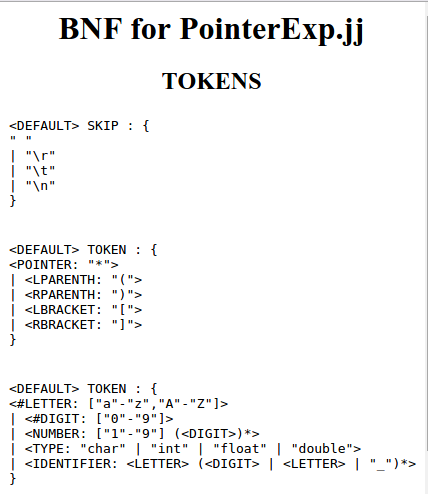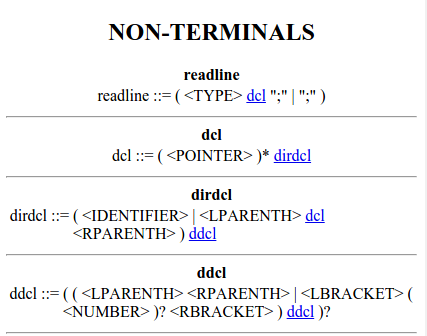### 5. CMM语法解析程序

CMM语言的文法规则如下：

```NEWLINE ::= "\r"? "\n"
WS      ::= " "|"\t"

IF      ::= "if"
ELSE    ::= "else"
WHILE   ::= "while"
WRITE   ::= "write"
INT     ::= "int"
REAL    ::= "real"
BOOL    ::= "bool"
TRUE    ::= "true"
FALSE   ::= "false"
PLUS    ::= "+"
MINUS   ::= "-"
TIMES   ::= "*"
DIVIDE  ::= "/"
ASSIGN  ::= "="

LT  ::= "<"
GT  ::= ">"
EQUAL   ::= "=="
NEQUAL  ::= "<>"

LPAREN  ::= "("
RPAREN  ::= ")"
LBRACE  ::= "{"
RBRACE      ::= "}"
LBRACKET    ::= "["
RBRACKET    ::= "]"

ROWCOMM     ::= "//"
LEFTCOMM    ::= "/*"
RIGHTCOMM   ::= "*/"
COMMA       ::= ","
SEMICOLON   ::= ";"

LETTER      ::= ["a"-"z"]|["A"-"Z"]
DIGIT       ::= ["0"-"9"]
ID          ::= <LETTER> ( ( <LETTER> | <DIGIT> | "_" ) * ( <LETTER> | <DIGIT> ) )?

INT_LITERAL ::= ["1"-"9"] (<DIGIT>)* | "0"
REAL_LITERAL::= <INT_LITERAL> ( "."( <INT_LITERAL> )+ )?

program     ::= statement

statement   ::= ( ifStmt | whileStmt | readStmt | writeStmt | assignStmt | declareStmt )+

ifStmt      ::= <IF> <LPAREN> condition <RPAREN> <LBRACE> statement <RBRACE> ( <ELSE> <LBRACE> statement <RBRACE> )?

whileStmt   ::= <WHILE> <LPAREN> condition <RPAREN> <LBRACE> statement <RBRACE>

writeStmt   ::= <WRITE> <LPAREN> expression <RPAREN> <SEMICOLON>

assignStmt  ::= <ID> [array] <ASSIGN> expression <SEMICOLON>

declareStmt ::= (<INT> | <REAL> | <BOOL>) [array] <ID> ( <COMMA> <ID>)* <SEMICOLON>

condition   ::= (expression compOp expression)|<TRUE>|<FALSE>

term        ::= factor (mulOp factor)*

compOp      ::= <LT> | <GT> | <EQUAL> | <NEQUAL>

mulOp       ::= <TIMES> | <DIVIDE>

factor      ::= <REAL_LITERAL> | <INT_LITERAL> | <ID> | <LPAREN> expression <RPAREN> | <ID> array

array       ::= <LBRACKET> ( <INT_LITERAL> | <ID> ) <RBRACKET>
```

```\$cd bin
java cmm.CMMGrammer         # read source code from keyboard
java cmm.CMMGrammer file    # read source code from file
```